## Tension force related to angular velocity

A question was posed in Physics class:

A toy “rocket-car” is attached to a metal rod that keeps the rocket moving in a horizontal circle on a frictionless table. The radius of the circle is 1.33 m, the mass of the rocket is 0.87 kg, and the rocket engine provides a thrust of 5.7 N. If the rocket starts from rest, how many revolutions will the rocket make before the tension in the rod equals 215 N?

Let’s pick out the important information from the equation:

Attached to a rod moving in a circle.
Radius (of the circle) = 1.33 m
Mass (of the rocket) = 0.87 kg
Thrust (of the engine) = 5.7 N
Final Tension Force = 215 N

Since it is going around in a circle on the table that is frictionless we can ignore gravity for this since it’s only 1 plane of motion.  The important thing to realize is that we need to find the relationship between tension on the arm and thrust of the engine which produces acceleration.  We know that as the object accelerates the tension increases.  We also know that if we can find the total distance traveled and divide it by the distance in one revolution, we’ll get the number of revolutions.

First, we will need to find out exactly how much the object is accelerating.  To get acceleration, we can work backwards since we have the force of thrust as 5.7 N.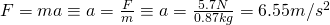Now, we need to figure out what the velocity of the object is when the tension force is 215 N.  To do this, we use the formula for angular force which can be changed to angular velocity: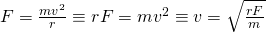This will give us our velocity.  We already know the variables needed, r is 1.33 m, F is the final tension force, 215 N and our mass is 0.87 kg.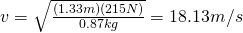Now we have the velocity.  Getting the circumference is the easy part: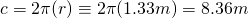Great!  Now we have two more steps.  The first is to use our handy formula that deals specifically with velocity, acceleration and distance values and obtain the total distance:We’re almost done.  Now that we have the total change in distance we can do simple division and we’ll get our answer: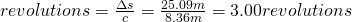And there you have it.  So the total times the object goes around the track before reaching 215 N of force on the arm is 3.

As always, please correct me if you see any problems.  Thanks!